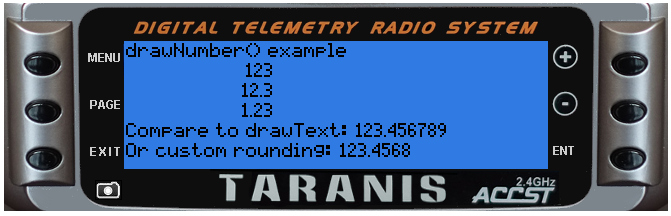## lcd.drawNumber(x, y, value [, flags])

Display a number at (x,y)

@status current Introduced in 2.0.0

#### Parameters

• `x,y` (positive numbers) starting coordinate

• `value` (number) value to display

• `flags` (unsigned number) drawing flags:

• `0 or not specified` normal representation
• `PREC1` display with one decimal place (number 386 is displayed as 38.6)
• `PREC2` display with tow decimal places (number 386 is displayed as 3.86)
• other general LCD flag also apply

none

### Examples

lcd/drawNumber-example

``````function round(num, decimals)
local mult = 10^(decimals or 0)
return math.floor(num * mult + 0.5) / mult
end

local function run(event)
lcd.clear()
lcd.drawText(1,1,"drawNumber() example", 0)
local myNumber = 123.456789
lcd.drawNumber(75, 11, myNumber, 0)
lcd.drawNumber(75, 21, myNumber, PREC1)
lcd.drawNumber(75, 31, myNumber, PREC2)
lcd.drawText(1, 41, "Compare to drawText: " .. myNumber, 0)
lcd.drawText(1, 51, "Or custom rounding: " .. round(myNumber, 4), 0)
end

return{run=run}
``````